# Partitioning a Segment in a Given Ratio

Suppose you have a line segment $\overline{PQ}$ on the coordinate plane, and you need to find the point on the segment $\frac{1}{3}$  of the way from $P$ to $Q$.

Let’s first take the easy case where $P$ is at the origin and line segment is a horizontal one.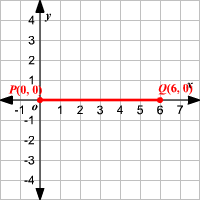The length of the line is $6$ units and the point on the segment $\frac{1}{3}$  of the way from $P$ to $Q$ would be $2$ units away from $P$, $4$ units away from $Q$ and would be at $\left(2,0\right)$.

Consider the case where the segment is not a horizontal or vertical line.The components of the directed segment $\overline{PQ}$ are $〈6,3〉$  and we need to find the point, say $X$ on the segment $\frac{1}{3}$  of the way from $P$ to $Q$.

Then, the components of the segment $\overline{PX}$ are $〈\left(\frac{1}{3}\right)\left(6\right),\left(\frac{1}{3}\right)\left(3\right)〉=〈2,1〉$.

Since the initial point of the segment is at origin, the coordinates of the point $X$ are given by $\left(0+2,0+1\right)=\left(2,1\right)$.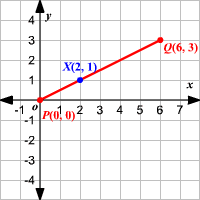Now let’s do a trickier problem, where neither $P$ nor $Q$ is at the origin.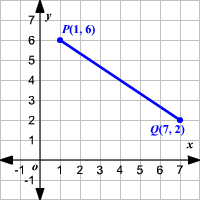Use the end points of the segment $\overline{PQ}$  to write the components of the directed segment.

$\begin{array}{l}〈\left({x}_{2}-{x}_{1}\right),\left({y}_{2}-{y}_{1}\right)〉=〈\left(7-1\right),\left(2-6\right)〉\\ \text{\hspace{0.17em}}\text{\hspace{0.17em}}\text{\hspace{0.17em}}\text{\hspace{0.17em}}\text{\hspace{0.17em}}\text{\hspace{0.17em}}\text{\hspace{0.17em}}\text{\hspace{0.17em}}\text{\hspace{0.17em}}\text{\hspace{0.17em}}\text{\hspace{0.17em}}\text{\hspace{0.17em}}\text{\hspace{0.17em}}\text{\hspace{0.17em}}\text{\hspace{0.17em}}\text{\hspace{0.17em}}\text{\hspace{0.17em}}\text{\hspace{0.17em}}\text{\hspace{0.17em}}\text{\hspace{0.17em}}\text{\hspace{0.17em}}\text{\hspace{0.17em}}\text{\hspace{0.17em}}\text{\hspace{0.17em}}\text{\hspace{0.17em}}\text{\hspace{0.17em}}\text{\hspace{0.17em}}\text{\hspace{0.17em}}\text{\hspace{0.17em}}\text{\hspace{0.17em}}\text{\hspace{0.17em}}\text{\hspace{0.17em}}\text{\hspace{0.17em}}\text{\hspace{0.17em}}\text{\hspace{0.17em}}\text{\hspace{0.17em}}\text{\hspace{0.17em}}\text{\hspace{0.17em}}\text{\hspace{0.17em}}\text{\hspace{0.17em}}\text{\hspace{0.17em}}\text{\hspace{0.17em}}\text{\hspace{0.17em}}\text{\hspace{0.17em}}=〈6,-4〉\end{array}$

Now in a similar way, the components of the segment $\overline{PX}$  where $X$ is a point on the segment $\frac{1}{3}$  of the way from $P$ to $Q$are $〈\left(\frac{1}{3}\right)\left(6\right),\left(\frac{1}{3}\right)\left(-4\right)〉=〈2,-1.25〉$.

To find the coordinates of the point $X$ add the components of the segment $\overline{PX}$  to the coordinates of the initial point $P$.

So, the coordinates of the point $X$ are $\left(1+2,6-1.25\right)=\left(3,4.75\right)$.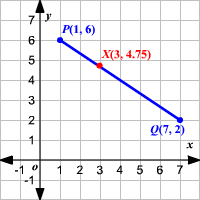Note that the resulting segments, $\overline{PX}$ and $\overline{XQ}$, have lengths in a ratio of $1:2$.

In general: what if you need to find a point on a line segment that divides it into two segments with lengths in a ratio $a:b$?

Consider the directed line segment $\overline{XY}$  with coordinates of the endpoints as $X\left({x}_{1},{y}_{1}\right)$  and $Y\left({x}_{2},{y}_{2}\right)$.

Suppose the point $Z$ divided the segment in the ratio $a:b$, then the point is $\frac{a}{a+b}$ of the way from $X$ to $Y$.

So, generalizing the method we have, the components of the segment $\overline{XZ}$ are $〈\left(\frac{a}{a+b}\left({x}_{2}-{x}_{1}\right)\right),\left(\frac{a}{a+b}\left({y}_{2}-{y}_{1}\right)\right)〉$.

Then, the $X$-coordinate of the point $Z$ is

$\begin{array}{l}{x}_{1}+\frac{a}{a+b}\left({x}_{2}-{x}_{1}\right)=\frac{{x}_{1}\left(a+b\right)+a\left({x}_{2}-{x}_{1}\right)}{a+b}\\ \text{\hspace{0.17em}}\text{\hspace{0.17em}}\text{\hspace{0.17em}}\text{\hspace{0.17em}}\text{\hspace{0.17em}}\text{\hspace{0.17em}}\text{\hspace{0.17em}}\text{\hspace{0.17em}}\text{\hspace{0.17em}}\text{\hspace{0.17em}}\text{\hspace{0.17em}}\text{\hspace{0.17em}}\text{\hspace{0.17em}}\text{\hspace{0.17em}}\text{\hspace{0.17em}}\text{\hspace{0.17em}}\text{\hspace{0.17em}}\text{\hspace{0.17em}}\text{\hspace{0.17em}}\text{\hspace{0.17em}}\text{\hspace{0.17em}}\text{\hspace{0.17em}}\text{\hspace{0.17em}}\text{\hspace{0.17em}}\text{\hspace{0.17em}}\text{\hspace{0.17em}}\text{\hspace{0.17em}}\text{\hspace{0.17em}}\text{\hspace{0.17em}}\text{\hspace{0.17em}}\text{\hspace{0.17em}}\text{\hspace{0.17em}}\text{\hspace{0.17em}}\text{\hspace{0.17em}}\text{\hspace{0.17em}}\text{\hspace{0.17em}}\text{\hspace{0.17em}}\text{\hspace{0.17em}}\text{\hspace{0.17em}}\text{\hspace{0.17em}}=\frac{b{x}_{1}+a{x}_{2}}{a+b}\end{array}$.

Similarly, the $Y$-coordinate is

$\begin{array}{l}{y}_{1}+\frac{a}{a+b}\left({y}_{2}-{y}_{1}\right)=\frac{{y}_{1}\left(a+b\right)+a\left({y}_{2}-{y}_{1}\right)}{a+b}\\ \text{\hspace{0.17em}}\text{\hspace{0.17em}}\text{\hspace{0.17em}}\text{\hspace{0.17em}}\text{\hspace{0.17em}}\text{\hspace{0.17em}}\text{\hspace{0.17em}}\text{\hspace{0.17em}}\text{\hspace{0.17em}}\text{\hspace{0.17em}}\text{\hspace{0.17em}}\text{\hspace{0.17em}}\text{\hspace{0.17em}}\text{\hspace{0.17em}}\text{\hspace{0.17em}}\text{\hspace{0.17em}}\text{\hspace{0.17em}}\text{\hspace{0.17em}}\text{\hspace{0.17em}}\text{\hspace{0.17em}}\text{\hspace{0.17em}}\text{\hspace{0.17em}}\text{\hspace{0.17em}}\text{\hspace{0.17em}}\text{\hspace{0.17em}}\text{\hspace{0.17em}}\text{\hspace{0.17em}}\text{\hspace{0.17em}}\text{\hspace{0.17em}}\text{\hspace{0.17em}}\text{\hspace{0.17em}}\text{\hspace{0.17em}}\text{\hspace{0.17em}}\text{\hspace{0.17em}}\text{\hspace{0.17em}}\text{\hspace{0.17em}}\text{\hspace{0.17em}}\text{\hspace{0.17em}}\text{\hspace{0.17em}}\text{\hspace{0.17em}}=\frac{b{y}_{1}+a{y}_{2}}{a+b}\end{array}$.

Therefore, the coordinates of the point $Z$ are $\left(\frac{b{x}_{1}+a{x}_{2}}{a+b},\frac{b{y}_{1}+a{y}_{2}}{a+b}\right)$.

Example 1:

Find the coordinates of the point that divides the directed line segment $\overline{MN}$ with the coordinates of endpoints at $M\left(-4,0\right)$  and $M\left(0,4\right)$ in the ratio $3:1$?

Let $L$ be the point that divides $\overline{MN}$  in the ratio $3:1$.

Here, $\left({x}_{1},{y}_{1}\right)=\left(-4,0\right),\left({x}_{2},{y}_{2}\right)=\left(0,4\right)$ and $a:b=3:1$.

Substitute in the formula. The coordinates of $L$ are

$\left(\frac{1\left(-4\right)+3\left(0\right)}{3+1},\frac{1\left(0\right)+3\left(4\right)}{3+1}\right)$.

Simplify.

$\left(\frac{-4+0}{4},\frac{0+12}{4}\right)=\left(-1,3\right)$

Therefore, the point $L\left(-1,3\right)$  divides $\overline{MN}$  in the ratio $3:1$.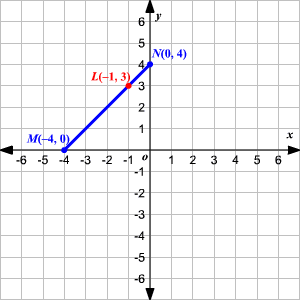Example 2:

What are the coordinates of the point that divides the directed line segment $\overline{AB}$  in the ratio $2:3$?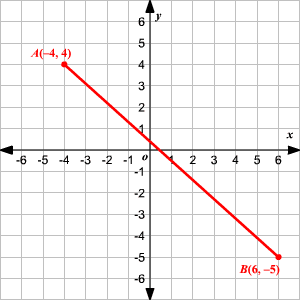Let $C$ be the point that divides $\overline{AB}$  in the ratio $2:3$.

Here, $\left({x}_{1},{y}_{1}\right)=\left(-4,4\right),\left({x}_{2},{y}_{2}\right)=\left(6,-5\right)$ and $a:b=2:3$.

Substitute in the formula. The coordinates of $C$ are

$\left(\frac{3\left(-4\right)+2\left(6\right)}{5},\frac{3\left(4\right)+2\left(-5\right)}{5}\right)$.

Simplify.

$\begin{array}{l}\left(\frac{-12+12}{5},\frac{12-10}{5}\right)=\left(0,\frac{2}{5}\right)\\ \text{\hspace{0.17em}}\text{\hspace{0.17em}}\text{\hspace{0.17em}}\text{\hspace{0.17em}}\text{\hspace{0.17em}}\text{\hspace{0.17em}}\text{\hspace{0.17em}}\text{\hspace{0.17em}}\text{\hspace{0.17em}}\text{\hspace{0.17em}}\text{\hspace{0.17em}}\text{\hspace{0.17em}}\text{\hspace{0.17em}}\text{\hspace{0.17em}}\text{\hspace{0.17em}}\text{\hspace{0.17em}}\text{\hspace{0.17em}}\text{\hspace{0.17em}}\text{\hspace{0.17em}}\text{\hspace{0.17em}}\text{\hspace{0.17em}}\text{\hspace{0.17em}}\text{\hspace{0.17em}}\text{\hspace{0.17em}}\text{\hspace{0.17em}}\text{\hspace{0.17em}}\text{\hspace{0.17em}}\text{\hspace{0.17em}}\text{\hspace{0.17em}}\text{\hspace{0.17em}}\text{\hspace{0.17em}}\text{\hspace{0.17em}}\text{\hspace{0.17em}}\text{\hspace{0.17em}}\text{\hspace{0.17em}}\text{\hspace{0.17em}}\text{\hspace{0.17em}}\text{\hspace{0.17em}}\text{\hspace{0.17em}}\text{\hspace{0.17em}}\text{\hspace{0.17em}}\text{\hspace{0.17em}}\text{\hspace{0.17em}}\text{\hspace{0.17em}}=\left(0,0.4\right)\end{array}$

Therefore, the point $C\left(0,0.4\right)$  divides $\overline{AB}$  in the ratio $2:3$.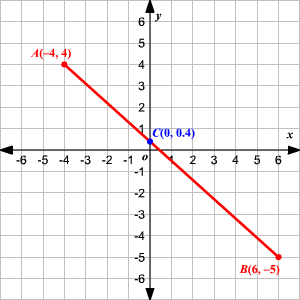You can note that the Midpoint Formula is a special case of this formula when $a=b=1$.